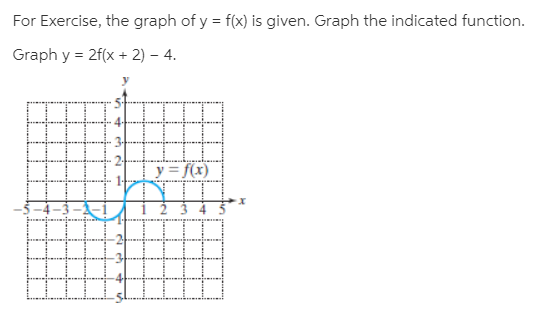# For Exercise, the graph of y = f(x) is given. Graph the indicated function. Graph y = 2f(x + 2) – 4. y = f(x) स

Questionhelp_outlineImage TranscriptioncloseFor Exercise, the graph of y = f(x) is given. Graph the indicated function. Graph y = 2f(x + 2) – 4. y = f(x) स fullscreen